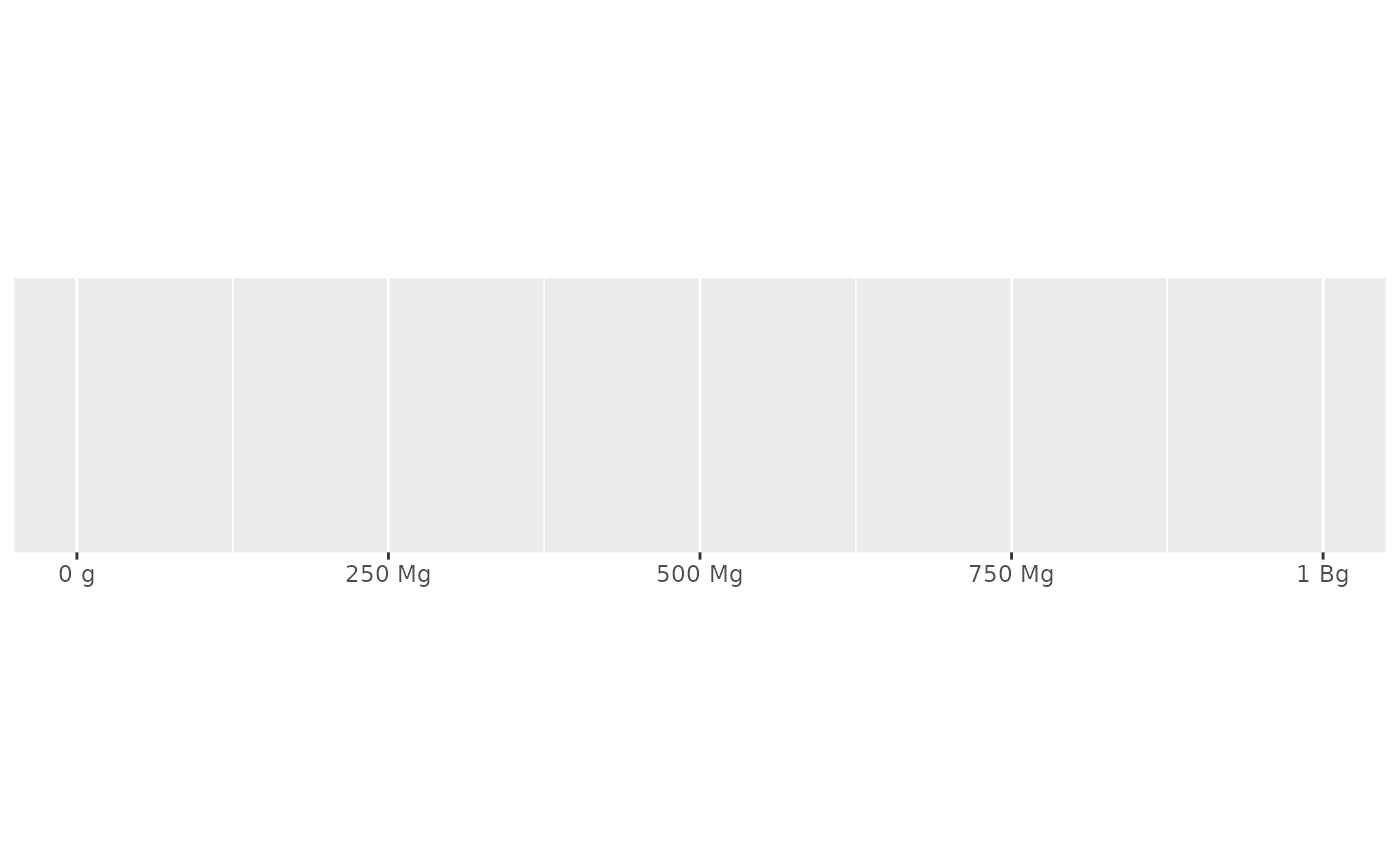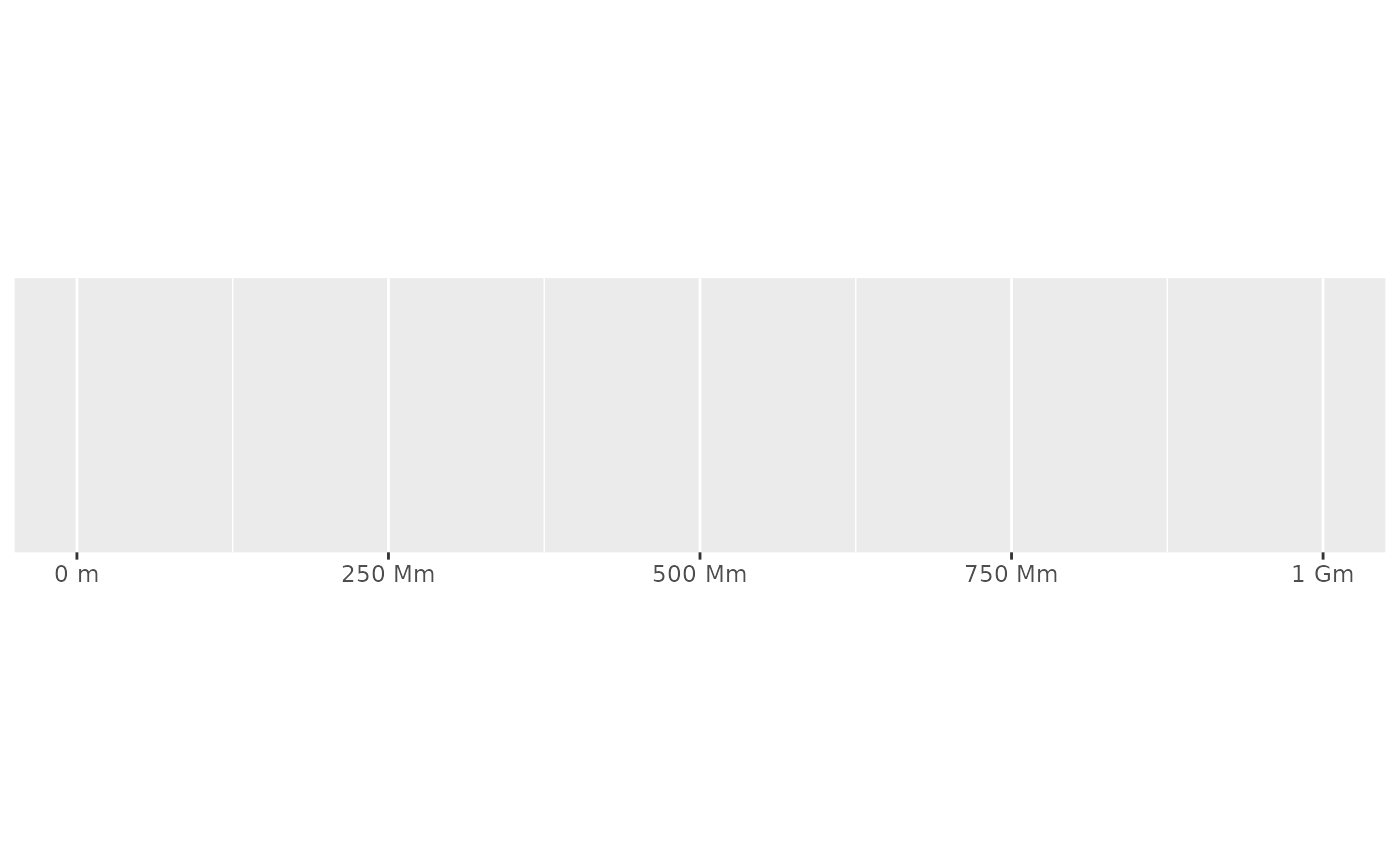label_number_si() automatically adds the most suitable SI prefix and scales the values appropriately. For example, values greater than 1000 gain a "k" prefix (abbreviated from "kilo-") and are scaled by 1/1000. See Metric Prefix on Wikipedia for more details.

label_number_si(unit, accuracy = NULL, scale = 1, ...)

## Arguments

unit Unit of measurement (e.g. "m" for meter, the SI unit of length). A number to round to. Use (e.g.) 0.01 to show 2 decimal places of precision. If NULL, the default, uses a heuristic that should ensure breaks have the minimum number of digits needed to show the difference between adjacent values. Applied to rescaled data. A scaling factor: x will be multiplied by scale before formatting. This is useful if the underlying data is already using an SI prefix. Other arguments passed on to base::format().

## Value

All label_() functions return a "labelling" function, i.e. a function that takes a vector x and returns a character vector of length(x) giving a label for each input value.

Labelling functions are designed to be used with the labels argument of ggplot2 scales. The examples demonstrate their use with x scales, but they work similarly for all scales, including those that generate legends rather than axes.

## See also

Other labels for continuous scales: label_bytes(), label_dollar(), label_number_auto(), label_ordinal(), label_parse(), label_percent(), label_pvalue(), label_scientific()

Other labels for log scales: label_bytes(), label_scientific()

## Examples

demo_continuous(c(1, 1000), labels = label_number_si("m"))
#> scale_x_continuous(labels = label_number_si("m"))demo_log10(c(1, 1e9), breaks = log_breaks(10), labels = label_number_si("m"))
#> scale_x_log10(breaks = log_breaks(10), labels = label_number_si("m"))demo_log10(c(1e-9, 1), breaks = log_breaks(10), labels = label_number_si("g"))
#> scale_x_log10(breaks = log_breaks(10), labels = label_number_si("g"))# use scale when data already uses SI prefix (e.g. stored in kg)
kg <- label_number_si("g", scale = 1e3)
demo_log10(c(1e-9, 1), breaks = log_breaks(10), labels = kg)
#> scale_x_log10(breaks = log_breaks(10), labels = kg)